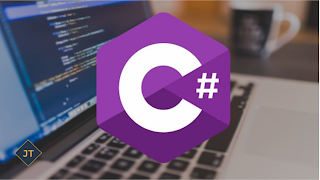Factorial in C#

Factorial of a number is obtained from the result of multiplying a series of descending natural numbers. Factorial of n is the product of all positive descending integers. Factorial of n is denoted by n!.

Example:
```5! = 5*4*3*2*1 = 120
6! = 6*5*4*3*2*1 = 720```
Here, 5! is pronounced as "5 factorial", it is also called "5 bang" or "5 shriek".
The factorial is normally used in Combinations and Permutations (mathematics).

3 Different ways to calculate factorial in C#

Using For Loop:
```using System;
using System.Collections.Generic;
using System.Linq;
using System.Text;
namespace factorial
{
class Program
{
static void Main(string[] args)
{
int i, number, fact;
Console.WriteLine("Enter the Number");
number = int.Parse(Console.ReadLine());
fact = number;
for (i = number - 1; i >= 1; i--)
{
fact = fact * i;
}
Console.WriteLine("\nFactorial of Given Number is: "+fact);
Console.ReadLine();
}
}
}```
Output:
```Enter the Number: 5
Factorial of Given Number is: 120```

Using Recursion:
```class Program
{
static void Main(string[] args)
{
int number;
Console.WriteLine("Enter the Number");
number = int.Parse(Console.ReadLine());
double fact;
fact = factorial_Recursion(number);
Console.WriteLine("\nFactorial of Given Number is: " + fact);
Console.ReadLine();
}
public static double factorial_Recursion(int number)
{
if (number == 1)
return 1;
else
return number * factorial_Recursion(number - 1);
}
}```
Output:
```Enter the Number: 8
Factorial of Given Number is: 40320```

Using While Loop:
```class Program
{
static void Main(string[] args)
{
int number;
Console.WriteLine("Enter the Number");
number = int.Parse(Console.ReadLine());
double fact;
fact = factorial_Recursion(number);
Console.WriteLine("\nFactorial of Given Number is: " + fact);
Console.ReadLine();
}
public static double factorial_Recursion(int number)
{
if (number == 1)
return 1;
else
return number * factorial_Recursion(number - 1);
}
}```
Output:
```Enter the Number: 4
Factorial of Given Number is: 24```

Hope It will clear about the Factorial in C#. Still have any question regarding this, you can add the comments on below Disqus Forum.